Processing ......FreeComputerBooks.com Links to Free Computer, Mathematics, Technical Books all over the World

Discrete Mathematics for Computer Science
Top Free Python Books 🌠 - 100% Free or Open Source!
• Title Discrete Mathematics for Computer Science
• Author(s) Jean H. Gallier
• Publisher: Springer; 2011 edition (January 25, 2011)
• Hardcover/Paperback 477 pages
• eBook PDF, PostScript, and other formats
• Language: English
• ASIN: 1441980466
• ISBN-13: 978-1441980465Book Description

This book gives an introduction to discrete mathematics for beginning undergraduates. One of original features of this book is that it begins with a presentation of the rules of logic as used in mathematics. Many examples of formal and informal proofs are given.

With this logical framework firmly in place, the book describes the major axioms of set theory and introduces the natural numbers. The rest of the book is more standard. It deals with functions and relations, directed and undirected graphs, and an introduction to combinatorics.

There is a section on public key cryptography and RSA, with complete proofs of Fermat's little theorem and the correctness of the RSA scheme, as well as explicit algorithms to perform modular arithmetic. The last chapter provides more graph theory. Eulerian and Hamiltonian cycles are discussed. Then, we study flows and tensions and state and prove the max flow min-cut theorem.

We also discuss matchings, covering, bipartite graphs.

The book is highly illustrated and each chapter ends with a list of problems of varying difficulty. Undergraduates in mathematics and computer science will find this book useful.

• Jean H. Gallier is a researcher in computational logic at the University of Pennsylvania, where he holds appointments in the Computer and Information Science Department and the Department of Mathematics.
Reviews, Ratings, and Recommendations: Related Book Categories: Read and Download Links:Similar Books:
•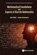Mathematical Foundations and Aspects of Discrete Mathematics

This is a book about discrete mathematics which also discusses mathematical reasoning and logic. It offers an introduction to discrete mathematics for undergraduate students in computer science and mathematics.

•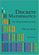Discrete Mathematics: An Open Introduction (Oscar Levin)

This is a gentle introduction to discrete mathematics. Four main topics are covered: counting, sequences, logic, and graph theory. Along the way proofs are introduced, including proofs by contradiction, proofs by induction, and combinatorial proofs.

•Discrete Structures for Computer Science: Counting, Recursion

This book provides a broad introduction to some of the most fascinating and beautiful areas of discrete mathematical structures with examples in logic, applications of the principle of inclusion and exclusion and finally the pigeonhole principal.

•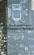Applied Discrete Structures, Fundamentals (Al Doerr, et al)

This book contains most of the fundamental concepts taught in a one semester course in discrete mathematics which is a required course for students in Computer Science, Mathematics and Information Technology.

•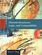Discrete Structures, Logic, and Computability (James L. Hein)

This book contains programming experiments that are designed to reinforce the learning of discrete mathematics, logic, and computability, provides introductions to those ideas and techniques to understand and practice the art and science of computing.

•A Spiral Workbook for Discrete Mathematics (Harris Kwong)

This is a book on discrete mathematics with an emphasis on motivation. It explains and clarifies the unwritten conventions in mathematics, and guides the students through a detailed discussion on how a proof is revised from its draft to a final polished form.

•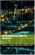Mathematics for Computer Scientists (Gareth J. Janacek, et al)

The aim of this book is to present some the basic mathematics that is needed by computer scientists. The reader is not expected to be a mathematician and we hope will find what follows useful.

•Lists, Decisions and Graphs - With an Introduction to Probability

In this book, four basic areas of discrete mathematics are presented: Counting and Listing (Unit CL), Functions (Unit Fn), Decision Trees and Recursion (Unit DT), and Basic Concepts in Graph Theory (Unit GT).

•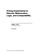Prolog Experiments in Discrete Math, Logic, and Computability

This book contains programming experiments that are designed to reinforce the learning of discrete mathematics, logic, and computability. The Prolog programming language is the tool used for the experiments in this book.

•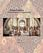Isomorphism - Mathematics of Programming (Xinyu Liu)

This book introduces the mathematics behind computer programming. It intents to tell: programming is isomorphic to mathematics. Just like in art and music, there are interesting stories and mathematicians behind the great minds.

•Discrete Mathematics for Computer Science Students

This book gives thorough coverage to topics that have great importance to computer scientists and provides a motivating computer science example for each math topic. Contents: Counting; Cryptography and Number Theory; Reflections on Logic and Proof.

•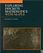Exploring Discrete Mathematics With Maple (Kenneth H. Rosen)

This is is a supplement in discrete mathematics to concentrate on the computational aspects of the computer algebra system Maple. Detailed instructions for the use of Maple are included in an introductory chapter and in each subsequent chapter.

Book Categories
 :All CategoriesTop Free BooksRecent BooksMiscellaneous BooksComputer EngineeringComputer LanguagesComputer ScienceData Science/DatabasesJava and Java EE (J2EE)Linux and UnixMathematicsMicrosoft and .NETMobile ComputingNetworking and CommunicationsSoftware EngineeringSpecial TopicsWeb Programming
Other Categories